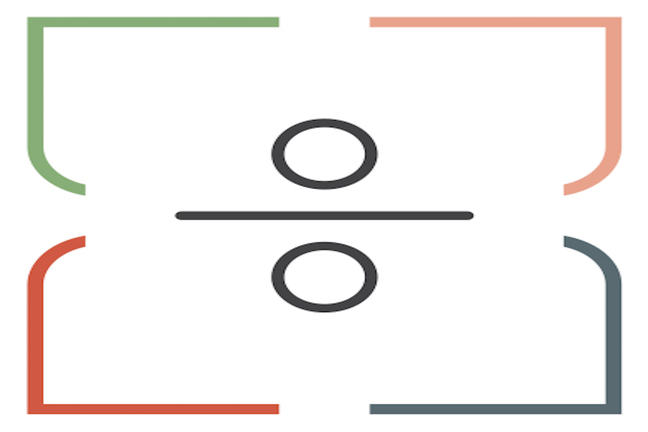## Want to keep learning?

This content is taken from the Davidson Institute of Science Education at the Weizmann Institute of Science's online course, Maths Puzzles: Cryptarithms, Symbologies and Secret Codes. Join the course to learn more.
1.15

## Davidson Institute of Science Education at the Weizmann Institute of Science# Tests of divisibility and more hints

Some multiplication rules can really help us when we get stuck on a cryptarithm. Here are some rules you should know before taking the ‘plunge’ into the more difficult problems…

• A number is divisible by 2 if it’s unit’s digit is an even number.
• A number is divisible by 3 if the sum of its digits is divisible by 3.
• A number is divisible by 5 if the number ends with a 0 or a 5.
• A number is divisible by 6 if it’s an even number and the sum of its digits is divisible by 3.
• A number is divisible by 9 if the sum of its digits is divisible by 9.
• A number is divisible by 11 when the difference between the sum of the digits in the even positions (looking at the number from left to right) and the sum of the digits in the odd positions is divisible by 11.

### Examples

The number 43242543 is divisible by 9 and by 3 because the digit-sum of the number: 4+3+2+4+2+5+4+3=27 is divisible both by 3 and by 9.

Is the number 1352467928 divisible by 11?

The sum of the digits in the even spots: 3+2+6+9+8=28

The sum of the digits in the odd spots: 1+5+4+7+2=19

Since 28-19=9 and 9 is not divisible by 11 the whole number is not divisible by 11.

We can ‘fix’ the number to be divisible by 11 if we can ‘fix’ the difference to be, i.e. 11. This can be done by adding 2 to one of the digits in the even spots. Let’s change the number 3 to 5. We get: 1552467928. This number is divisible by 11:

The sum of the digits in the even spots: 5+2+6+9+8=30

The sum of the digits in the odd spots: 1+5+4+7+2=19

Since 30-19=11 and 11 is divisible by 11 the whole number, 1552467928, is divisible by 11.

Here are some useful multiplication rules that can help solve cryptarithms:

When you multiply a number by: The units digit in the product will be:
0 0
1 0,1,2,3,4,5,6,7,8,9
2 0,2,4,6,8
3 0,1,2,3,4,5,6,7,8,9
4 0,2,4,6,8
5 0,5
6 0,2,4,6,8
7 0,1,2,3,4,5,6,7,8,9
8 0,2,4,6,8
9 0,1,2,3,4,5,6,7,8,9